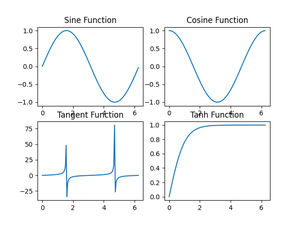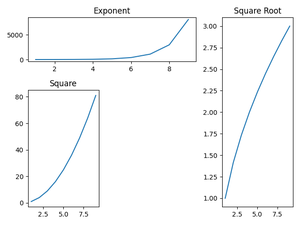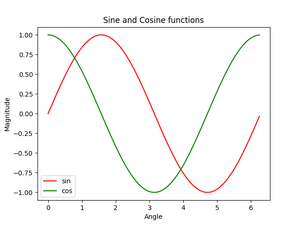# Plot multiple plots in Matplotlib

• Difficulty Level : Medium
• Last Updated : 03 Jan, 2021

Prerequisites: Matplotlib

In Matplotlib, we can draw multiple graphs in a single plot in two ways. One is by using subplot() function and other by superimposition of second graph on the first i.e, all graphs will appear on the same plot. We will look into both the ways one by one.

Attention geek! Strengthen your foundations with the Python Programming Foundation Course and learn the basics.

To begin with, your interview preparations Enhance your Data Structures concepts with the Python DS Course. And to begin with your Machine Learning Journey, join the Machine Learning - Basic Level Course

## Multiple Plots using subplot () Function

A subplot () function is a wrapper function which allows the programmer to plot more than one graph in a single figure by just calling it once.

Syntax: matplotlib.pyplot.subplots(nrows=1, ncols=1, sharex=False, sharey=False, squeeze=True, subplot_kw=None, gridspec_kw=None, **fig_kw)

Parameters:

1. nrows, ncols:  These gives the number of rows and columns  respectively. Also, it must be noted that both these parameters are optional and the default value is 1.
2. sharex, sharey:  These parameters specify about the properties that are shared among a and y axis.Possible values for them can be, row, col, none or default value which is False.
3. squeeze: This parameter is a boolean value specified, which asks the programmer whether to squeeze out, meaning remove the extra dimension from the array. It has a default value False.
4. subplot_kw: This parameters allow us to add keywords to each subplot and its default value is None.
5. gridspec_kw: This allows us to add grids on each subplot and has a default value of None.
6. **fig_kw: This allows us to pass any other additional keyword argument to the function call and has a default value of None.

Example :

## Python3

 `# importing libraries``import` `matplotlib.pyplot as plt``import` `numpy as np``import` `math`` ` `# Get the angles from 0 to 2 pie (360 degree) in narray object``X ``=` `np.arange(``0``, math.pi``*``2``, ``0.05``)`` ` `# Using built-in trigonometric function we can directly plot``# the given cosine wave for the given angles``Y1 ``=` `np.sin(X)``Y2 ``=` `np.cos(X)``Y3 ``=` `np.tan(X)``Y4 ``=` `np.tanh(X)`` ` `# Initialise the subplot function using number of rows and columns``figure, axis ``=` `plt.subplots(``2``, ``2``)`` ` `# For Sine Function``axis[``0``, ``0``].plot(X, Y1)``axis[``0``, ``0``].set_title(``"Sine Function"``)`` ` `# For Cosine Function``axis[``0``, ``1``].plot(X, Y2)``axis[``0``, ``1``].set_title(``"Cosine Function"``)`` ` `# For Tangent Function``axis[``1``, ``0``].plot(X, Y3)``axis[``1``, ``0``].set_title(``"Tangent Function"``)`` ` `# For Tanh Function``axis[``1``, ``1``].plot(X, Y4)``axis[``1``, ``1``].set_title(``"Tanh Function"``)`` ` `# Combine all the operations and display``plt.show()`

OutputMultiple plots using subplot() function

In Matplotlib, there is another function very similar to subplot which is subplot2grid (). It is same almost same as subplot function but provides more flexibility to arrange the plot objects according to the need of the programmer.

This function is written as follows:

Syntax: matplotlib.pyplot.subplot2grid(shape, loc, rowspan=1, colspan=1, fig=None, **kwargs)

Parameter:

1. shape
This parameter is a sequence of two integer values which tells the shape of the grid for which we need to place the axes. The first entry is for row, whereas the second entry is for column.
2. loc
Like shape parameter, even Ioc is a sequence of 2 integer values, where first entry remains for the row and the second is for column to place axis within grid.
3. rowspan
This parameter takes integer value and the number which indicates the number of rows for the axis to span to or increase towards right side.
4. colspan
This parameter takes integer value and the number which indicates the number of columns for the axis to span to or increase the length downwards.
5. fig
This is an optional parameter and takes Figure to place axis in. It defaults to current figure.
6. **kwargs
This allows us to pass any other additional keyword argument to the function call and has a default value of None.

Example :

## Python3

 `# Importing libraries``import` `matplotlib.pyplot as plt``import` `numpy as np``import` `math`` ` `# Placing the plots in the plane``plot1 ``=` `plt.subplot2grid((``3``, ``3``), (``0``, ``0``), colspan``=``2``)``plot2 ``=` `plt.subplot2grid((``3``, ``3``), (``0``, ``2``), rowspan``=``3``, colspan``=``2``)``plot3 ``=` `plt.subplot2grid((``3``, ``3``), (``1``, ``0``), rowspan``=``2``)`` ` `# Using Numpy to create an array x``x ``=` `np.arange(``1``, ``10``)`` ` `# Plot for square root``plot2.plot(x, x``*``*``0.5``)``plot2.set_title(``'Square Root'``)`` ` `# Plot for exponent``plot1.plot(x, np.exp(x))``plot1.set_title(``'Exponent'``)`` ` `# Plot for Square``plot3.plot(x, x``*``x)``plot.set_title(``'Square'``)`` ` `# Packing all the plots and displaying them``plt.tight_layout()``plt.show()`

OutputMultiple Plots using subplot2grid() function

## Plotting in same plot

We have now learnt about plotting multiple graphs using subplot and subplot2grid function of Matplotlib library. As mentioned earlier, we will now have a look at plotting multiple curves by superimposing them. In this method we do not use any special function instead we directly plot the curves one above other and try to set the scale.

Example :

## Python3

 `# Importing libraries``import` `matplotlib.pyplot as plt``import` `numpy as np``import` `math`` ` `# Using Numpy to create an array X``X ``=` `np.arange(``0``, math.pi``*``2``, ``0.05``)`` ` `# Assign variables to the y axis part of the curve``y ``=` `np.sin(X)``z ``=` `np.cos(X)`` ` `# Plotting both the curves simultaneously``plt.plot(X, y, color``=``'r'``, label``=``'sin'``)``plt.plot(X, z, color``=``'g'``, label``=``'cos'``)`` ` `# Naming the x-axis, y-axis and the whole graph``plt.xlabel(``"Angle"``)``plt.ylabel(``"Magnitude"``)``plt.title(``"Sine and Cosine functions"``)`` ` `# Adding legend, which helps us recognize the curve according to it's color``plt.legend()`` ` `# To load the display window``plt.show()`

Outputsine and cosine function curve in one graph

My Personal Notes arrow_drop_up